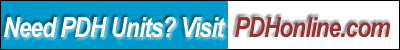Print this page

Robert B. Wilcox, P.E.

Course Outline

This course will cover spreadsheet based analysis for general purpose engineering use. It will focus on using basic calculations, formulas and graphs within Microsoft Excel ™. Several sample problems will be modeled, accompanied by sample spreadsheets which may be downloaded and used for understanding the examples. One powerful feature of the spreadsheet lies in its capability to rapidly compare the effects of changing parameters on the solution to an engineering problem. In this way, the sensitivity of the solution to each variable may be determined. Design examples will be given in the areas of stress analysis, heat transfer and engineering economics. Additional topics will include decision making logic, and goal-seeking (a form of automated trial and error). Course Requires Microsoft Excel™ or similar program, (such as Open Office Calc - an open source free program available as part of the Open Office Suite, see http://www.openoffice.com/)

• Prerequisites and Required Software
• The Basic Elements of a Spreadsheet
• Entering Data
• Basic Operators
• Entering a Formula
• Goal Seeking
• Introduction to Math Functions
• Creating a Chart Embedded in the Worksheet
• Statistical Functions
• Financial Functions
• Logical Expressions
• A Data Table Example
• Sorting Data
• Filtering Data
• Importing Data from the Web into a Spreadsheet
• Formatting Basics

The course includes a multiple-choice quiz at the end, which is designed to enhance the understanding of the course materials.

Learning Objective

At the conclusion of this course, the student will:

• Understand the basic elements of a spreadsheet;
• Comprehend absolute and relative references;
• Know the difference between a workbook and a worksheet;
• Be able to enter a simple formula;
• Understand basic operators and the order of operations;
• Learn what a function is, and the syntax of a function;
• Learn to apply basic math functions like sin, cosine, and square root;
• Be able to apply basic logical operations;
• Learn to use basic statistical functions;
• Comprehend the use of basic financial functions;
• Be able to construct a logical expression using operators like IF, AND and OR;
• Learn what goal seeking is and how to use it to solve for the optimal answer to a problem;
• Learn how to set up a data table;
• Understand how to sort data;
• Comprehend basic filtering of data;
• Learn how to import data from a web-based source into a spread sheet;
• Understand basic chart functionality;
• Know how to format cells;
• Apply the basic concepts to a parametric study of sizing a beam;
• Be able to set up a problem with multiple variables and solve for the best solution;
• Understand how to solve an engineering economics problem involving internal rate of return;
• Be able to apply the goalseek function; and
• See how embedded graphs can be used as an immediate feedback tool in the analysis.

Intended Audience

This course is intended for engineers or architects who want to learn how to use spreadsheets effectively, primarily for analysis of physical, real-world problems.

Benefit to Attendees

The attendee will obtain the basics required for setting up a spreadsheet based analysis, and be introduced to some features they might not ordinarily use, such as data filtering, logical tests, and goal seeking solutions.

Course Introduction

Engineers and architects frequently find themselves performing repetitive calculations on similar problems. Using spreadsheets as a working tool provides a means for a powerful semi-automatic parametric design approach. By using variables and recording the calculations in a spreadsheet, it is possible to rapidly and inexpensively evaluate several alternatives before finalizing the design. If a similar design situation then arises in the future, the problem is already framed and it becomes simply a mater of changing the values of the design variables to achieve a satisfactory new solution.

This course will cover the basics from square one, moving along quickly, progressing through to the design examples, and presenting some perhaps lesser known but very powerful features involving data sorting and filtering, logical decision making, and taking some of the mystery out of setting up the formulas in the spreadsheet based design.

Course Content

The course content is in a PDF file (500 KB) Excel Spreadsheet Basics for Engineers.

Course Summary

Excel is a powerful analysis tool for the engineer or architect, with capabilities which go far beyond simply keeping track of data. Calculations may be standardized, optimized, reiterated, and compared rapidly and with graphical feedback. Most basic mathematical, financial and logical functions used in everyday calculations can easily be set up in a spreadsheet, saving time and providing a benchmark for future reference.